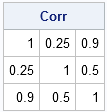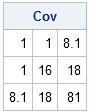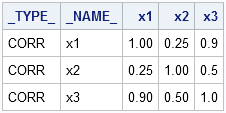I have previously blogged about how to convert a covariance matrix into a correlation matrix in SAS (and the other way around). However, I still get questions about it, perhaps because my previous post demonstrated more than one way to accomplish each transformation.

To eliminate all confusion, the following SAS/IML program implements a function that converts a covariance matrix into the associated correlation matrix. For an explanation of why this works, see my previous article.

```proc iml; /* convert a covariance matrix, S, to a correlation matrix */ start Cov2Corr(S); D = sqrt(vecdiag(S)); return( S / D` / D ); /* divide columns, then divide rows */ finish;   S = {1.0 1.0 8.1, /* test it */ 1.0 16.0 18.0, 8.1 18.0 81.0 }; Corr = Cov2Corr(S); print Corr;```There are many covariance matrices that produce the same correlation matrix, therefore the inverse operation (which produces a covariance matrix from a correlation matrix) is not uniquely defined. However, if you specify the standard deviations for each variable, you can write a SAS/IML function that converts a correlation matrix to a covariance matrix:

```/* R = correlation matrix sd = (vector of) standard deviations for each variable Return covariance matrix with sd##2 on the diagonal */ start Corr2Cov(R, sd); std = colvec(sd); return( std` # R #std ); finish;   /* convert correlation matrix to covariance matrix */ sd = {1 4 9}; /* std devs = sqrt(vecdiag(S)) */ Cov = Corr2Cov(Corr, sd); print Cov;```### New in SAS/IML 12.3 software

Update: As of SAS/IML 12.3, these functions are now built into SAS/IML software. See the documentation for the CORR2COV function and the COV2CORR function.

### Converting a covariance matrix to a correlation matrix with SAS/STAT software

You can, of course, use the DATA step to convert a covariance matrix to a correlation matrix (and the other way around), but here's a neat trick that you can do in SAS/STAT software: the FACTOR procedure can read a covariance matrix (technically, a TYPE=COV data set) and write the corresponding correlation matrix to a SAS data set, as shown in the following example.

```/* define covariance matrix as TYPE=COV data set (lower triangular) */ data cov(type=cov); _type_='COV'; input x1-x3; cards; 1 . . 1 16 . 8.1 18 81 ; run; /* convert covariance matrix to correlation matrix */ proc factor data=cov outstat=outstat noprint; run; proc print data=outstat noobs; where _TYPE_="CORR"; run;```Does anyone know of a slick trick that converts a correlation matrix (and standard deviations) into a covariance matrix without using SAS/IML software? Post your solution in the comments.

ShareDistinguished Researcher in Computational Statistics

Rick Wicklin, PhD, is a distinguished researcher in computational statistics at SAS and is a principal developer of SAS/IML software. His areas of expertise include computational statistics, simulation, statistical graphics, and modern methods in statistical data analysis. Rick is author of the books Statistical Programming with SAS/IML Software and Simulating Data with SAS.

1.Love this blog!

I have a question about singular covariance matrices! I seem to have run into one...and my understanding is that this should not be the case. I am considering a 189x189 covariance matrix produced by a major ratings agency. I tried to use this covariance matrix to produce a correlation matrix, but the covariance matrix appears to be non-invertible, or singular, or have a determinant = 0.

Does your IML text or your simulation text address this scenario?

Thanks

Brian

•Yes, this can happen. It occurs when a variable (column of a matrix) is a linear combination of the other variables (columns). For example:

x = shape(1:12, 4);
cov = cov(x);
d = det(cov);
print d;

The workaround is to identify the collinearities in the data. Alternatively, run PROC PRINCOMP or PROC VARCLUS on the data to obtain a lower dimensional set of variables that nevertheless captures most (or all) of the variance.